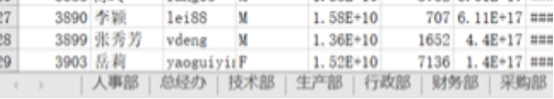# python如何实现工作表合并？1、获取所有的sheet表

2、转化列表推导式

3、调用pandas库

4、使用concat()方法

5、进行sheet表连接

```import pandas as pd
import os
curpath = os.path.dirname(__file__)
filename = os.path.join(curpath, 'example_split.xlsx')
savefilename = os.path.join(curpath, 'example_split_1.xlsx')
print(type(df))
dfs=[y for x,y in df.items()]
writer = pd.ExcelWriter(savefilename,engine='openpyxl', mode='a')
df2=pd.concat(dfs)
print(df2)
df2.to_excel(writer,'汇总表',index=False)
writer.save()```